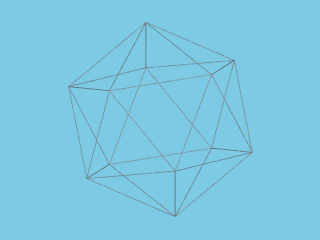The four regular non-convex polyhedra

click on an image to enlarge...

It is known that the five Platonic polyhedra are the only regular convex polyhedra. A polyhedron, considered as a solid is convex if and only if the line segment between any two points of the polyhedron belongs entirely to the solid. However, if we admit a polyhedron to be non-convex, there exist four more types of regular polyhedra!

The four regular non-convex polyhedra are known as the Kepler-Poinsot Polyhedra. Two of them were described by Johannes Kepler in 1619 as being regular, although the objects themselves certainly were known earlier. One of them appears on a 16th century drawing by Jamnitzer and the other on a 15th century mosaic on the floor of the San Marco in Venice. The other two were described by Louis Poinsot in 1809 but at least one of them appears on a drawing by the same Jamnitzer. In 1810 the French mathematician Augustin-Louis Cauchy proved that the five Platonic and the four Kepler-Poinsot polyhedra are the only possible regular polyhedra.

All four Kepler-Poinsot polyhedra can be constructed starting from a regular dodecahedron or icosahedron. It' my purpose to demonstrate a possible construction for each of them.

The two Kepler polyhedra

1. The Small Stellated DodecahedronBy extending the edges of a face of a regular dodecahedron we obtain a pentagram. Proceeding in this way for all twelve faces we obtain a non-convex polyhedron. This polyhedron is called a small stellated dodecahedron. It has 12 faces (pentagrams), 12 vertices and 30 edges. Five faces meet in each vertex. The small stellated dodecahedron can be constructed by putting appropriated five-sided pyramids upon the faces of the given dodecahedron. Each face of those pyramids is a "golden triangle" (see ex. Golden section...) . This can be helpful in case of a material construction of the solid. The vertices of the small stellated dodecahedron are also the vertices of an icosahedron.

2. The Great Stellated DodecahedronWe start with a regular icosahedron and consider one of it's vertices. The other endpoints of the five edges starting from the choosen vertex are the vertices of a pentagon. We extend the sides of this pentagon to obtain a pentagram. As the icosahedron has 12 vertices we obtain 12 pentagrams. They are the faces of a non-convex polyhedron, called a great stellated dodecahedron. It has 12 faces (pentagrams), 20 vertices and 30 edges. Three faces meet in each vertex. The great stellated dodecahedron can be constructed by putting appropriated three-sided pyramids upon the faces of the given icoshedron. Each face of those pyramids is a "golden triangle" (see ex. Golden section...) . This can be helpful in case of a material construction of the solid. The vertices of the great stellated dodecahedron are also the vertices of a dodecahedron.

The two Poinsot polyhedra

1. The Great DodecahedronThe start is very similar as in the case of the great stellated dodecahedron. We start with a regular icosahedron and again we consider one of it's vertices. The other endpoints of the five edges starting from the choosen vertex are the vertices of a pentagon. As the icosahedron has 12 vertices we obtain 12 pentagons. All of them are situated inside the icosahedron. They are the faces of a regular non-convex polyhedron, called a great dodecahedron. It has 12 faces (pentagons), 12 vertices and 30 edges. Three faces meet in each vertex. The great dodecahedron has the same vertices and edges as the icosahedron we started with.

2. The Great IcosahedronWe consider the vertices of a regular icosahedron. If we connect each vertex with his five "nearest neighbours" we obtain the edges of the icosahedron. But each vertex also has five "next nearest neighbours". Five combinations of the vertex choosen and two of his "next nearest neighbours" form equilateral triangles inside the icosahedron. As each vertex belongs to five triangles there are 20 equilateral triangles. They are the faces of a regular non-convex polyhedron, called a great icosahedron. It has 20 faces (triangles), 12 vertices and 30 edges. Five faces meet in each vertex. The great icosahedron has the same vertices as the icosahedron we started with.

Last update: October 24, 2001.